# Unity3D Shader系列之Compute Shader基础及图像灰度化

2020年11月22日 作者 老王

# 1.什么是Compute Shader

Compute Shader也是Shader中的一种，也运行在GPU上。它和我们平时说的顶点、片元着色器不同地方在于，Compute Shader不经过渲染流水线，而是用于通用计算。什么是通用计算呢？就是把之前在CPU的计算挪到GPU这边来，基于GPU强大的并行计算能力，与通过CPU计算相比能极大地缩短计算时间。使用GPU进行通用计算又叫GPGPU(General Purpose GPU Programming)编程。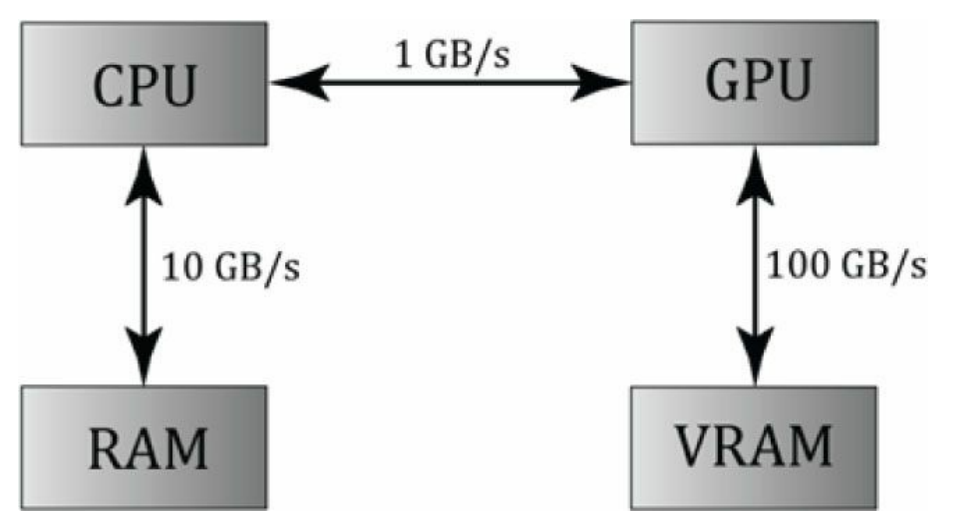Unity中Compute Shader是用什么语言写的呢？标准的DX11 HLSL

# 2.Compute Shader语法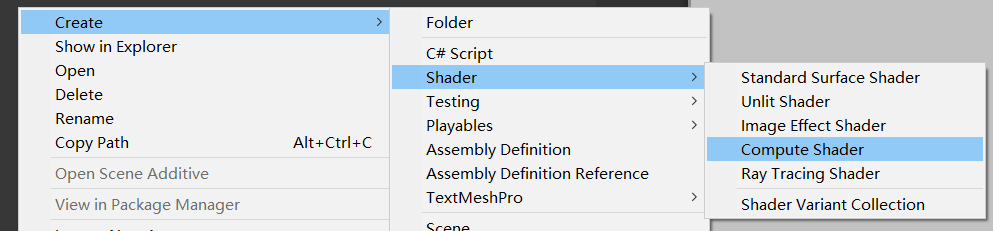// 申明内核
#pragma kernel CSMain

// 定义变量
Texture2D inputTex;
RWTexture2D<float4> outputTex;

// 定义线程组内线程的数量
[numthreads(8,8,1)]
void CSMain (uint3 id : SV_DispatchThreadID)
{
float r = inputTex[id.xy].r;
float g = inputTex[id.xy].g;
float b = inputTex[id.xy].b;
float a = inputTex[id.xy].a;

float gray = r * 0.299 + g * 0.587 + b * 0.114;
outputTex[id.xy] = float4(gray, gray, gray, a);
}

## 2.1 #pragma kernel

#pragma kernel CSMain

FindKernel的参数只有一个，即Compute Shader中内核名(由#pragma kernal指定的)，返回值为该内核的索引。

// 参数为内核名(由#pragma kernal指定的)，返回值为该内核的索引。
int kernal = cs.FindKernel("CSMain");

## 2.2 Compute Shader中的变量

Compute Shader语言是HLSL，所以HLSL支持的变量其都支持。

### 2.2.1 标量

bool 32位整数值用于存放逻辑值true和false
int 32位有符号整数
uint 32位无符号整数
half 16位浮点数(仅提供用于向后兼容)
float 32位浮点数
double 64位浮点数

### 2.2.2 向量

3种申明向量的方式。
1.使用类似模板的形式来描述

vector<float, 4> vec1;  // 向量vec1包含4个float元素
vector<int, 2> vec2;    // 向量vec2包含2个int元素

2.直接在基本类型后面加上数字

float4 vec1;    // 向量vec1包含4个float元素
int3 vec2;      // 向量vec2包含3个int元素

3.使用vector本身则表示为一种包含4个float元素的类型**

vector vec1;    // 向量vec1包含4个float元素

float2 vec0 = {0.0f, 1.0f};
float3 vec1 = float3(0.0f, 0.1f, 0.2f);
float4 vec2 = float4(vec1, 1.0f);

float4 vec0 = {1.0f, 2.0f, 3.0f, 0.0f};
float f0 = vec0.x;  // 1.0f
float f1 = vec0.g;  // 2.0f
float f2 = vec0; // 3.0f
vec0.a = 4.0f;      // 4.0f

float4 vec0 = {1.0f, 2.0f, 3.0f, 4.0f};
float3 vec1 = vec0.xyz;     // (1.0f, 2.0f, 3.0f)
float2 vec2 = vec0.rg;      // (1.0f, 2.0f)
float4 vec3 = vec0.zzxy;    // (4.0f, 4.0f, 1.0f, 2.0f)
vec3.wxyz = vec3;           // (2.0f, 4.0f, 4.0f, 1.0f)
vec3.yw = ve1.zz;           // (2.0f, 3.0f, 4.0f, 3.0f)

### 2.2.3 矩阵(matrix)

float1x1 float1x2 float1x3 float1x4
float2x1 float2x2 float2x3 float2x4
float3x1 float3x2 float3x3 float3x4
float4x1 float4x2 float4x3 float4x4

matrix<float, 2, 2> mat1;   // float2x2

matrix mat1;    // float4x4

float2x2 mat1 = {
1.0f, 2.0f, // 第一行
3.0f, 4.0f  // 第二行
};
float3x3 TBN = float3x3(T, B, N); // T, B, N都是float3

matrix M;
// ...

float f0 = M._m00;      // 第一行第一列元素(索引从0开始)
float f1 = M._12;       // 第一行第二列元素(索引从1开始)
float f2 = M;     // 第一行第二列元素(索引从0开始)
float4 f3 = M._11_12;   // Swizzles

matrix M;
vector v = {1.0f, 2.0f, 3.0f, 4.0f};
// ...

M = v;               // 矩阵的第一行被赋值为向量v
M._m11 = v;          // 等价于M = v;和M._22 = v;
M._12_21 = M._21_12;    // 交换M和M

float4 vec0 = 2.0f * float4(1.0f, 2.0f, 3.0f, 4.0f);    //(2.0f, 4.0f, 6.0f, 8.0f)
float4 vec1 = vec0 * float4(1.0f, 0.2f, 0.1f, 0.0f);    //(2.0f, 0.8f, 0.6f, 0.0f)

### 2.2.4 数组

float M;
int p;
float3 v;   // 12个3D向量

## 2.2.5 StructuredBuffer

struct A
{
float4 vec;
};

struct B
{
int scalar;
float4 vec;
float4x4 mat;
float arr;
A a;
};

// 结构体的访问
B b;
b.vec = float4(1.0f, 2.0f, 3.0f, 4.0f);

StructuredBuffer、RWStructuredBuffer和ComputeBuffer如何使用见下面这个例子。
Compute Shader：

struct Vertex {
float3 pos;
float2 uv;
}

RWStructuredBuffer< Vertex > buffer;

C#:

// 定义与Compute Shader中结构体相同的结构体
struct Vertex
{
public Vector3 pos;         // c#中的Vector3对应Compute Shader中的float3可对应
public Vector2 uv;          // c#中的Vector2可对应float2
}

private void RunShader()
{
// 定义结构体数组
Vertex[] vertex = new Vertex;
Vertex[] result = new Vertex;

// 初始化vertex数据...

// 示例化一个ComuteBuffer，用于和Compute Shader的RWStructuredBuffer交互
// ComputeBuffer构造函数需指定“数组的长度”， “一个结构体所占用的字节数”
// 我们的结构体中一共5个float，一个float占4个字节，所以该结构体占20个字节
ComputeBuffer buffer = new ComputeBuffer(vertex.Length, 20);
// vertex的设置给ComputeBuffer
buffer.SetData(vertex);
// 加载ComputeShader
ComputeShader cs = Resources.Load<ComputeShader>("xxx");
// FindKernel("内核")，获取到内核Id
int kernel = cs.FindKernel("CSMain");
// 将buffer中的值赋值给Compute Shader中的buffer，将两者联系起来
cs.SetBuffer(kernel, "buffer", buffer);
// 执行kernal这个内核
cs.Dispatch(kernel, 8, 8, 1);
// 从Compute Shader中读取计算后的值，结果存在result中
buffer.GetData(result);
// 不再使用时释放内存
buffer.Dispose();
}

1.Compute Buffer实例化，需指定数组的长度，和数组中单个元素占用的字节数

ComputeBuffer buffer = new ComputeBuffer(vertex.Length, 20);

2.使用ComputeBuffer.SetData，传入一个数组，将该数组中的值拷贝到ComputeBuffer

// vertex的设置给ComputeBuffer
buffer.SetData(vertex);

3.使用ComputeShader.SetBuffer将buffer指定给Compute Shader中StructuredBuffer\RWStructuredBuffer，参数分别是内核名、Compute Shader中StructuredBuffer\RWStructuredBuffer的变量名、要传递的ComputeBuffer

// 将buffer中的值赋值给Compute Shader中的buffer，将两者联系起来
cs.SetBuffer(kernel, "buffer", buffer);

4.ComputeShader.Dispatch执行ComputeShader
Dispatch中的参数是什么意思？后面我们会讲。
5.最后从Compute Shader中读取计算后的结果，方法为ComputeBuffer.GetData

// 从Compute Shader中读取计算后的值，结果存在result中
buffer.GetData(result);

### 2.2.6 Texture

Texture2D< float4 > xx;                 // 只读
RWTexture2D< float4 > xx;               // 读写
RWTexture2D< float2 > xx;               // RG_int

Texture2d< float4 >对应c#中的Texture2D。
RWTexture2d< float4 >对应c#中的RenderTexture。

cs.SetTexture(kernal, "inputTex", inputTexture);

## 2.3 numthreads与Dispatch

Compute Shader利用了GPU的并行处理，这里的并行处理是指Compute Shader中的内核方法会被GPU中的多个线程同时运行。

但要注意这种处理是无序的随机的，并不一定是固定的处理顺序，例如不一定是从左到右挨个处理像素点。

### 2.3.1 numthreads

[numthreads(8,8,1)]
void CSMain (uint3 id : SV_DispatchThreadID)
{
// ...
}

numthreads它的作用是什么呢？它是用来指定线程组(Threads Group)中线程的数量。

numthreads后面指定了x，y，z三个方向各有多少个线程。画了个图，相信大家一看就明白了。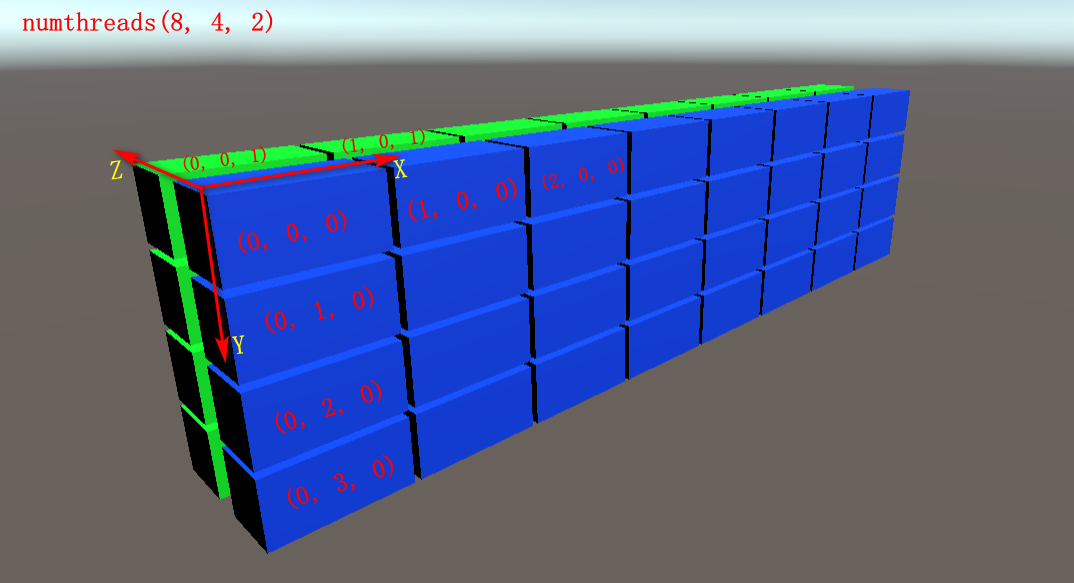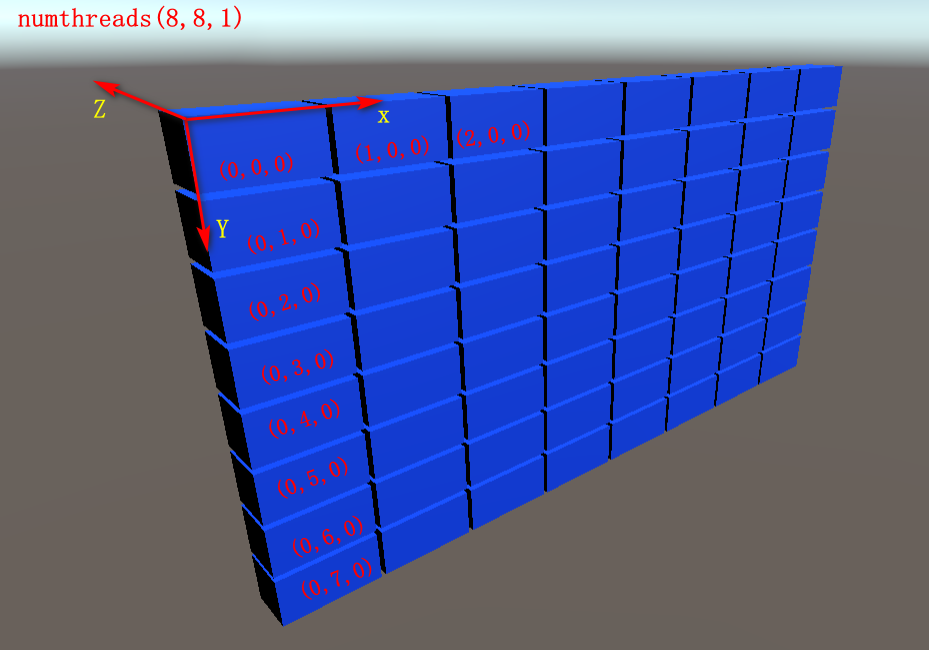### 2.3.3 numthreads中的xyz值何时最优

GPU一次Dispatch会调用64（AMD成为wavefront）或32（NVIDIA称为warp）个线程（这实际上是一种SIMD技术），所以，numThreads的乘积最好是这个值的整数倍。但是Mali不需要这种优化。此外，Metal可以通过API获取这个值。

numthreads的数值除了应该为32或64的整数倍外，也是有大小限制的，具体如下表所示。

Compute Shader Maximum Z Maximum Threads (XYZ)
cs_4_x 1 768
cs_5_0 64 1024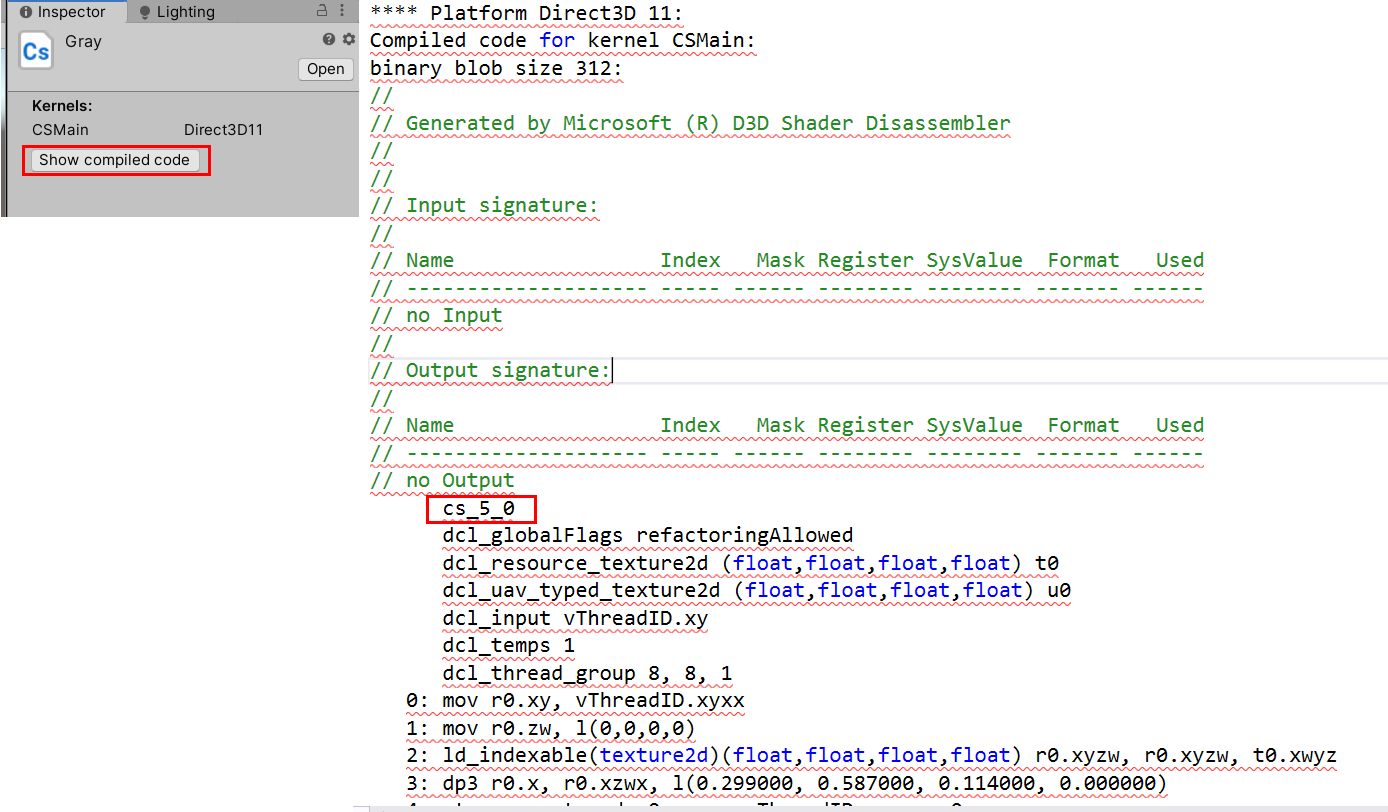### 2.3.4 Dispatch

ComputeShader.Dispatch用来指定有多少个线程组。
Dispatch用法如下，第一个参数为内核索引值，后面三个参数分别为x、y、z轴的线程组数量。

cs.Dispatch(kernal, 1024/8, 768/8, 1);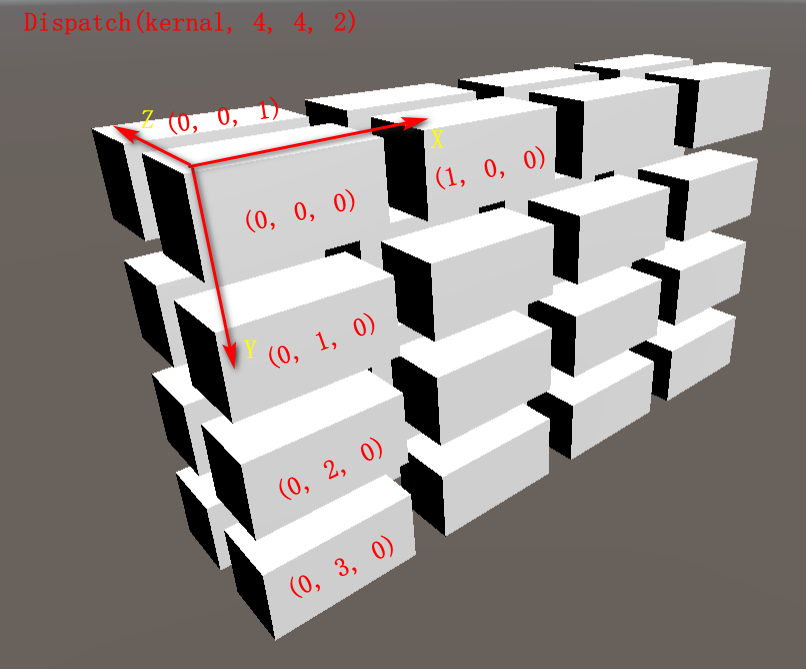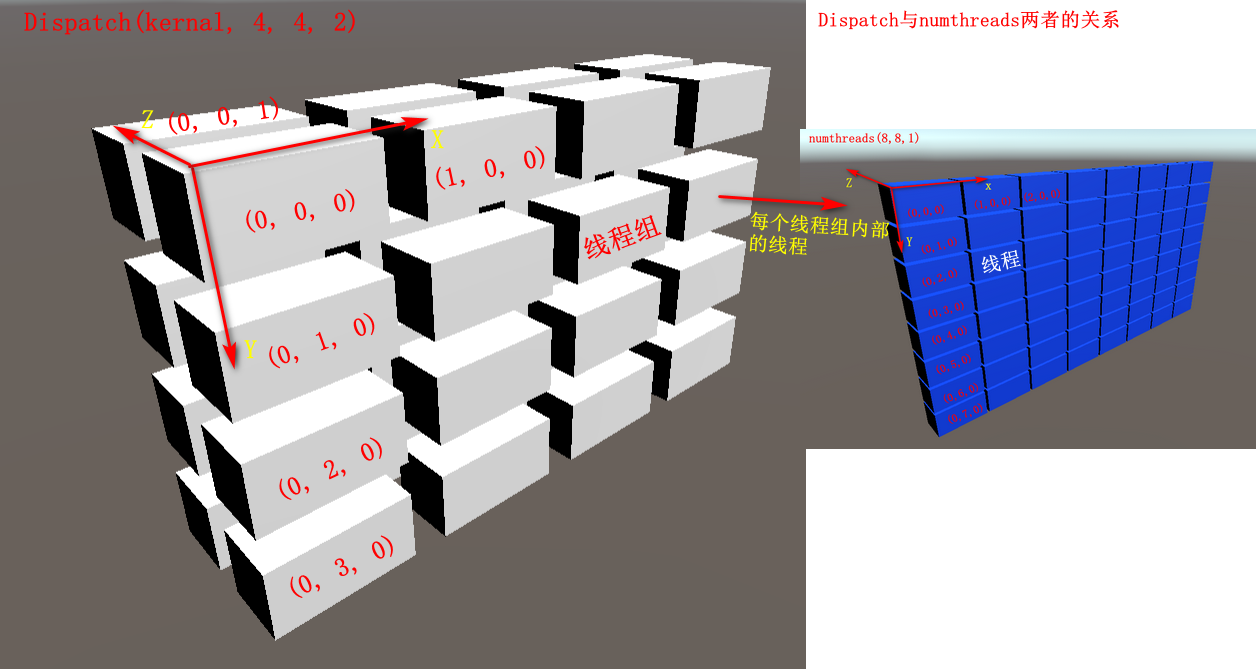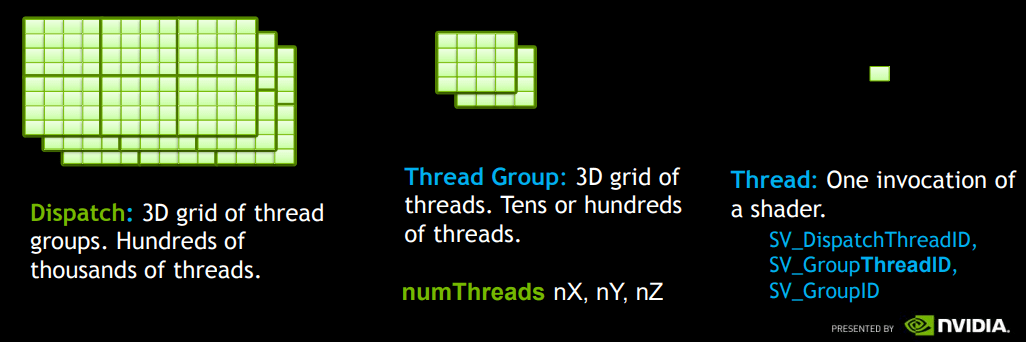A Thread Group 运行在一个GPU单元 （A single multiprocesser）,如果GPU有16个
multiprocesser，那么程序至少要分成16个 Thread Group使得每个multiprocesser都参与计算。

## 2.4 SV_DispatchThreadID

[numthreads(8,8,1)]
void CSMain (uint3 id : SV_DispatchThreadID)
{
// ...
}

SV_DispatchThreadID用标识当前线程在整个线程组中的位置，是一个三维向量。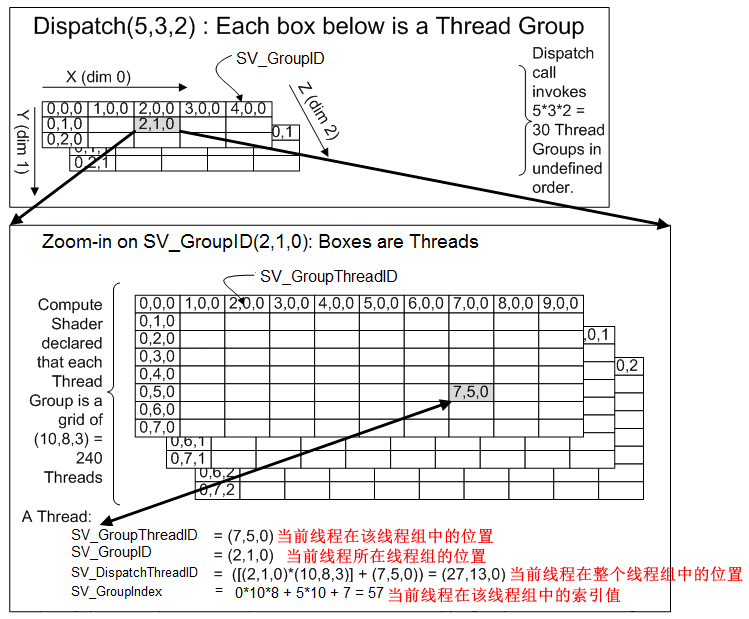SV_GroupThreadID 当前线程在该线程组中的位置
SV_GroupID 当前线程所在线程组的位置
SV_DispatchThreadID 当前线程在整个线程组中的位置
SV_GroupIndex 当前线程在该线程组中的索引值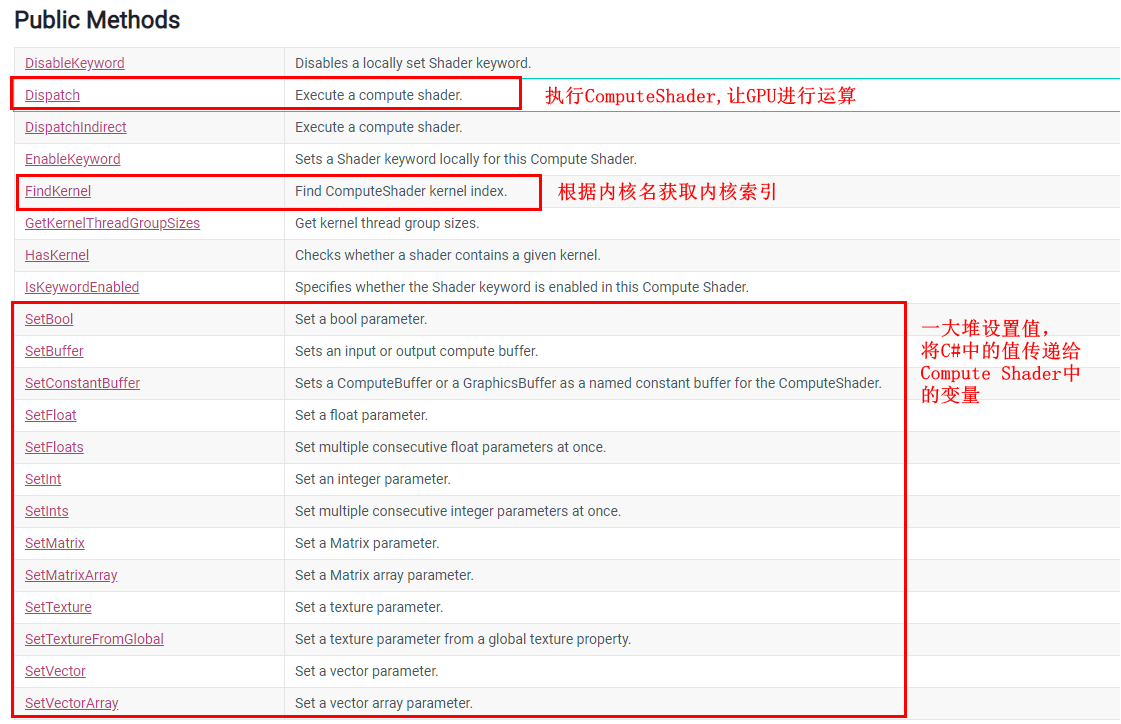# 3. Compute Shader灰度化图像

Compute Shader:

#pragma kernel CSMain

Texture2D<float4> inputTex;
RWTexture2D<float4> outputTex;

[numthreads(8,8,1)]
void CSMain (uint3 id : SV_DispatchThreadID)
{
float r = inputTex[id.xy].r;
float g = inputTex[id.xy].g;
float b = inputTex[id.xy].b;
float a = inputTex[id.xy].a;

float gray = dot(inputTex[id.xy].rgb, float3(0.299, 0.587, 0.114));
outputTex[id.xy] = float4(gray, gray, gray, a);
}

C#:

using UnityEngine;
using UnityEngine.UI;

public class GrayDemo : MonoBehaviour
{
private ComputeShader m_GrayComputeShader;

private RawImage m_GrayImg;

private void Start()
{
m_GrayImg = GameObject.Find("Canvas/RawImg-ComputeShader").GetComponent<RawImage>();

Texture2D inputTexture = Resources.Load<Texture2D>("flower_art_0EN071");

RenderTexture rt = new RenderTexture(inputTexture.width, inputTexture.height, 24);
rt.enableRandomWrite = true;
rt.Create();

m_GrayImg.texture = rt;
m_GrayImg.rectTransform.SetSizeWithCurrentAnchors(RectTransform.Axis.Horizontal, rt.width);
m_GrayImg.rectTransform.SetSizeWithCurrentAnchors(RectTransform.Axis.Vertical, rt.height);

m_GrayComputeShader = Resources.Load<ComputeShader>("ComputeShader/Gray");
int kernal = m_GrayComputeShader.FindKernel("CSMain");
m_GrayComputeShader.SetTexture(kernal, "inputTex", inputTexture);
m_GrayComputeShader.SetTexture(kernal, "outputTex", rt);

m_GrayComputeShader.Dispatch(kernal, 1024/8, 768/8, 1);
}
}